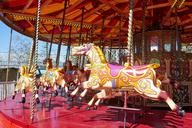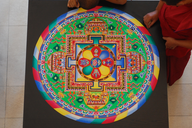## Visualizing Multiclass Classification ResultsIntroduction Visualizing the results of a binary classifier is already a challenge, but having more than two classes aggravates the matter considerably. Let’s say we have $k$ classes. Then for each observation, there is one correct prediction and $k-1$ possible incorrect prediction. Instead of a $2 \times 2$ confusion matrix, we have a $k^2$ possibilities. Instead of having two kinds of error, false positives and false negatives, we have $k(k-1)$ kinds of errors.